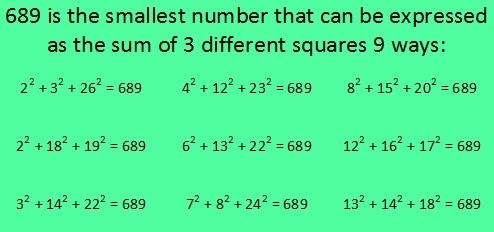# What Makes 689 Amazing?

689 is an amazing number for several reasons. I decided to make graphics to illustrate many of those ways. (689’s factoring information is at the end of this post.)

689 is the sum of consecutive prime numbers 227, 229, and 233.

Also 689 is the sum of the primes from 83 to 109. Do you know what those 7 prime numbers are?

OEIS.org informs us that 689 is the smallest number that can be expressed as the sum of three different square numbers NINE ways. I decided to figure out what those nine ways are and make this first graphic to share with you:Note: 614 can also be expressed as the sum of 3 squares 9 different ways, but one of those ways is 17² + 17² + 6² = 614, and that duplicates 17² in the same sum.

689 is the same number when it is turned upside down. Numbers with that characteristic are called Strobogrammatic numbers.689 BASE 10 isn’t a palindrome, but 373 BASE 14 is; note that 3(196) + 7(14) + 3(1) = 689

Both of 689’s prime factors have a remainder of 1 when divided by 4, so they are hypotenuses of Pythagorean triples. That fact also means 689 can be expressed as the sum of two square numbers TWO different ways, and it makes 689 the hypotenuse of FOUR Pythagorean triples.  Can you tell by looking at the graphic which two are primitive and which two aren’t?689 is the sum of consecutive numbers three different ways:

• 344 + 345 = 689; that’s 2 consecutive numbers.
• 47 + 48 + 49 + 50 + 51 + 52 + 53 + 54 + 55 + 56 + 57 + 58 + 59 = 689; that’s 13 consecutive numbers.
• 14 + 15 + 16 + 17 + 18 + 19 + 20 + 21 + 22 + 23 + 24 + 25 + 26 + 27 + 28 + 29 + 30 + 31 + 32 + 33 + 34 + 35 + 36 + 37 + 38 + 39 = 689; that’s 26 consecutive numbers.

Now you have a few reasons why 689 is an amazing number. 13 and 53 were part of some of those reasons so it shouldn’t surprise anyone to see 13 and 53 pop up in its factoring information, too:

——————————————————————————

• 689 is a composite number.
• Prime factorization: 689 = 13 x 53
• The exponents in the prime factorization are 1 and 1. Adding one to each and multiplying we get (1 + 1)(1 + 1) = 2 x 2 = 4. Therefore 689 has exactly 4 factors.
• Factors of 689: 1, 13, 53, 689
• Factor pairs: 689 = 1 x 689 or 13 x 53
• 689 has no square factors that allow its square root to be simplified. √689 ≈ 26.248809.